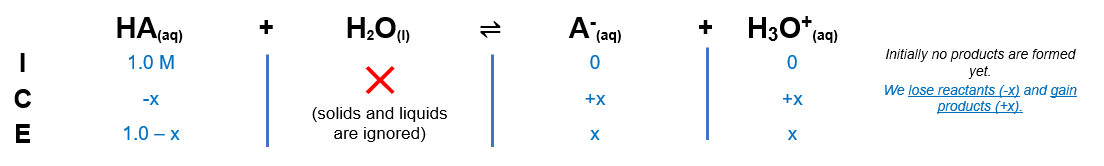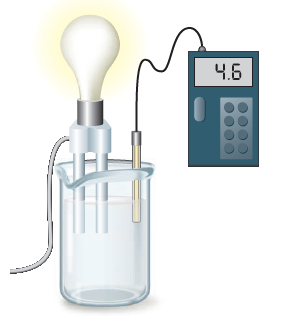# Problem: A solution is tested for pH and conductivity as pictured below:The solution contains one of the following substances: HCl, NaOH, NH4Cl, HCN, NH3, HF, or NaCN. If the solute concentration is about 1.0 M, what is the identity of the solute?

###### FREE Expert Solution

We can see that the reading is less than 7 so it means it is acidic.

However, it is also not that low so it must be a weak acid.

We can narrow down our options to:

NH4Cl, HCN and HF

Make an ICE chart:Recall:

Calculate x using pH:

Calculate Ka:

K= 6.31 x10-10

81% (26 ratings)###### Problem Details

A solution is tested for pH and conductivity as pictured below:

The solution contains one of the following substances:

HCl, NaOH, NH4Cl, HCN, NH3, HF, or NaCN. If the solute concentration is about 1.0 M, what is the identity of the solute?